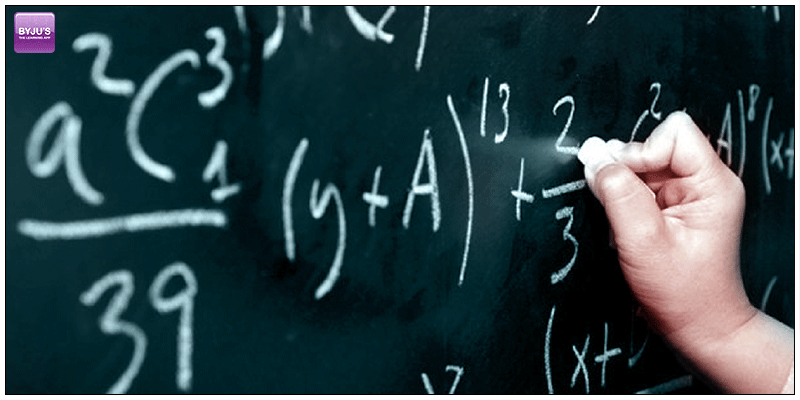# GMAT Quant: Algebra – Algebraic ExpressionsIn mathematics, an expression that is built from integer, variables, constants, joined by the algebraic operations, such as; exponentiation, addition, multiplication, subtraction, and division, etc.

## Algebraic Expressions Terminology

Terms and Factors

A term in an algebraic expression is an expression involving letters and/or numbers (called factors), multiplied together.

In the expression 5x, ‘x’ is the variable and 5 is the coefficient of the term.

Like Terms

Those terms which contains the same variables of same power is known as Like Terms.

$3x^{2}$ and $7x^{2}$ are like terms but $-8x^{2}$ and $5y^{2}$ are not.

Let us solve a problem to understand it properly.

Question: If $(x^{2} + 6x + 9) + 6 (x + 3) + 9 = 0$ Then the value of x =?

Solution: if $(x^{2} + 6x + 9) + 6 (x + 3) + 9 = 0$

$(x + 3)^{2} + 6 (x + 3) + 9 = 0$

Let’s assume (x + 3) = a

$(a)^{2} + 6a + 9 = 0$

$(a + 3)^{2} = 0$

a = -3

Since, a = x + 3

So, x + 3 = -3

x = -6

## Algebra (Word Problem)

Example 1: There are 43 boys in the class.  This is three more than four times the number of girls. How many girls are in the class?

Solution: Let ‘x’ be the number of girls in the class.

Therefore,

$4x + 3 = 43$

$4x = 43 – 3$

$x = \frac{43 – 3}{4}$

$x = 10$

Hence, there are 10 girls present in the class.

Example 2: Nazneen’s father is 5 times older than Nazneen and Nazneen is twice as old as her sister Jasmine. In two years time, the sum of their ages will be 58. How old is Nazneen now?

Let the present age of Nazneen is ‘x’

Then the Present Age of –

Nazneen = x

Jasmine = $\frac{1}{2}x$

Nazneen’s Father = 5x

In 2 years, their respective age will be

Nazneen = x + 2

Jasmine = $\frac{1}{2}x + 2$

Nazneen’s Father = 5x + 2

As, the sum of their age after 2 years is 58

Therefore,

$5x + 2 + x + 2 +\frac{1}{2}x + 2 = 58$

$5x + x +\frac{1}{2}x = 58 – (2 + 2 + 2)$

$6\frac{1}{2}x = 52$

$\Rightarrow x = 8$

Therefore the present age of Nazneen is 8 Years.